## Executive Summary

In order to demonstrate the principles of linear regression, in this project, I investigate two questions using the `mtcars` dataset:

1. Is an automatic or manual transmission better for MPG?

2. Quantify the MPG difference between automatic and manual transmissions.

These seemingly simple questions yield very different answers depending on how one chooses to model the given data. In this investigation, I’ll explore answers to these questions based on a number of different linear regression models.

• In a simple model considering only miles per gallon (`mpg`) and `transmission` (transformed from `am`), we expect a car with a manual transmission to gain an extra 7.24 +/- 3.60 miles per gallon more in fuel efficiency than a car with an automatic transmission.

• In a parallel slopes model predicting `mpg` from `transmission` and now the additional variable of weight (`wt`), we expect a car with a manual transmission to gain an extra -0.02 +/- 3.16 miles per gallon in fuel efficiency than a car with an automatic transmission, holding `wt` constant (or essentially no expected difference).

• In the same model but allowing for interaction between `wt` and `transmission`, the expected difference in `mpg` between automatic and manual transmission cars varies as `wt` changes. Holding `wt` constant, for cars greater than approximately 2800 lbs, we expect an automatic transmission car to receive higher `mpg`, whereas for a car lighter than 2800 lbs we would expect a manual transmission car to receive higher `mpg`.

• After exploring all possible variables for model inclusion, we settled upon a parsimonious model with three explanatory variables (`wt`, quarter mile time `qsec`, and `transmission`) able to explain 85% of the total variation in `mpg`. Under this model, holding `wt` and `qsec` constant, we expect cars with a manual transmission to get an extra 2.94 +/- 2.89 miles per gallon over cars with an automatic transmission.

Overall, we can conclude that a manual transmission does seem to be a better option than an automatic transmission in terms of miles per gallon, but the magnitude of this difference is tempered if we regress out the effects of a car’s weight and quarter mile time.

## Exploratory Data Analysis

``````data("mtcars")
library(ggplot2)
library(dplyr)
library(datasets)
library(knitr)
library(GGally)
library(car)``````

I will start by calculating summary statistics comparing cars with automatic and manual transmissions in terms of `mpg`. I’ll first create a new variable to make our labels more interpretable in plots.

``````# create a new column
mtcars\$transmission <- NA

# copy the data from the existing column into the new one
mtcars\$transmission <- mtcars\$am

# recode data to shorter labels, keeping factor order
mtcars\$transmission <- dplyr::recode(mtcars\$transmission,
`0` = 'Automatic', `1` = 'Manual')

# make it a factor variable
mtcars\$transmission <- as.factor(mtcars\$transmission)

# summary statistics table
table <- mtcars %>%
group_by(transmission) %>%
summarise(n = n(),
min = min(mpg),
q1 = quantile(mpg, 0.25),
median = median(mpg),
mean_mpg = mean(mpg),
q3 = quantile(mpg, 0.75),
max = max(mpg),
sd_mpg = sd(mpg))``````
transmission n min q1 median mean_mpg q3 max sd_mpg
Automatic 19 10.4 14.95 17.3 17.147 19.2 24.4 3.834
Manual 13 15.0 21.00 22.8 24.392 30.4 33.9 6.167

Then let’s examine a boxplot overlaid with a dotplot to visualize where the data lie in terms of `mpg` and `transmission`.

``````# plot mpg by transmission
ggplot(mtcars, aes(y = mpg, x = transmission)) +
geom_boxplot() +
geom_dotplot(binaxis = 'y', stackdir = 'center', fill = 'red') +
labs(x = "Transmission", y = "Miles Per Gallon (MPG)",
title = "Miles Per Gallon (MPG) by Transmission")``````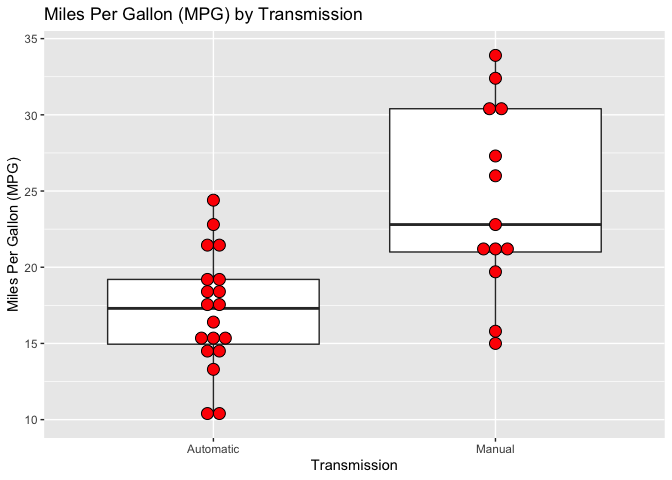The plot suggests that cars with manual transmissions tend to perform better in terms of `mpg`. Q1, the median, and Q3 are all higher for cars with manual transmissions. In fact, Q1 of manual transmission cars is greater than Q3 of automatic transmission cars.

However, the actual data points themselves show us that this is by no means always the case. As confirmed by the table, the variance among cars with manual transmissions is greater than those with automatic transmissions. There are examples of automatic cars in the dataset with higher `mpg` than manual cars.

Comparing histograms is another way to show the overlap in distributions despite manual transmissions having an advantage.

``````# mutate a mean mpg variable by group
mtcars <- mtcars %>%
group_by(transmission) %>%
mutate(mean_mpg = mean(mpg))

# comparative histogram
ggplot(mtcars, aes(x = mpg)) +
geom_histogram(binwidth = 1.5) +
facet_wrap(~ transmission, nrow = 2) +
geom_vline(aes(xintercept = mean_mpg), col = 'red') +
labs(x = "Miles Per Gallon (MPG)", y = "Frequency",
title = "Histograms of MPG by Transmission",
subtitle = "Group mean plotted in red")``````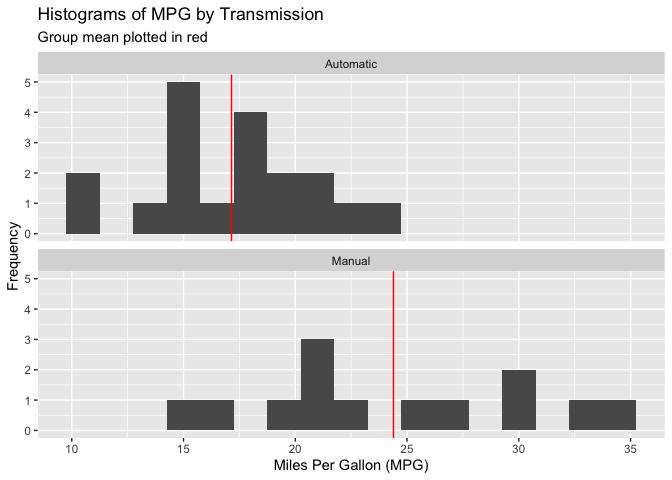Accordingly, I could try to answer the question of whether a manual transmission is “better” for `mpg` than an automatic transmission by creating a hypothesis test in order to determine whether this perceived advantage among manual transmission cars can be attributed to random chance. At the same time, by creating a confidence interval we will be able to quantify the MPG difference between automatic and manual transmissions (Question 2).

## Inference

### Null and Alternative Hypotheses

My null hypothesis is that the mean `mpg` for automatic transmission cars is equal to that of manual transmission cars. My alternative hypothesis is that the mean `mpg` for cars with a manual transmission is greater than that of cars with automatic transmissions.

### Assumptions

Before running such a test, one should evaluate to see if the data matches the required conditions to employ such a test.

• We require a bivariate explanatory variable, in this case `transmission`. As there are only two choices of transmission, this is clearly met.

• We require a continuous response variable, in this case `mpg`. This is also met as `mpg` is a numeric variable.

• Observations must be independent. We do not know how exactly Motor Trend magazine selected these cars for inclusion in the sample, but for this purpose, we can treat it as a random sample and assume independence.

• Data should have a normal distribution with the same variance in each group. Our normal quantile plot below and histograms above suggest that the data, while not heavily skewed, is not sufficiently normal.

``````# normal quantile plot
qqnorm(mtcars\$mpg)
qqline(mtcars\$mpg)``````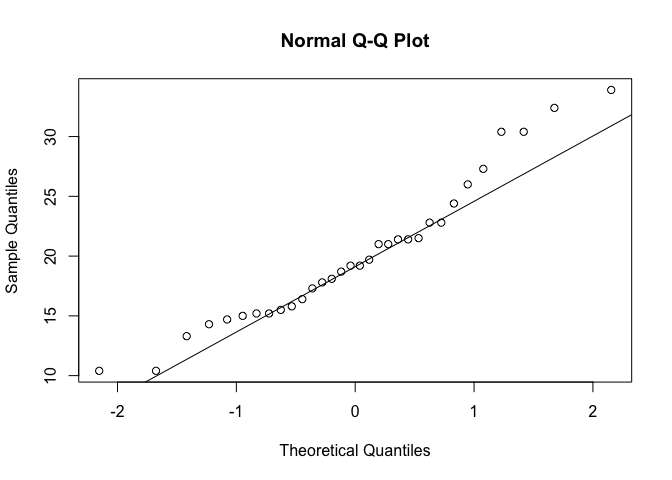However, the general rule of thumb is that sample sizes of more than 30 cross into the domain of the Central Limit Theorem. At a sample size of 32, this threshold is just barely met.

### Independent Sample T-Test

With these conditons more or less satisfied, let’s perform an independent sample, one-sided t-test to compare `mpg` of automatic and manual transmission cars, based on the null hypothesis above.

``````# filter data
mpg_manual <- mtcars[mtcars\$transmission == "Manual",]\$mpg
mpg_auto <- mtcars[mtcars\$transmission == "Automatic",]\$mpg

# perform t-test
t.test(mpg_manual, mpg_auto, var.equal = FALSE, alternative = 'greater')``````
``````##
##  Welch Two Sample t-test
##
## data:  mpg_manual and mpg_auto
## t = 3.7671, df = 18.332, p-value = 0.0006868
## alternative hypothesis: true difference in means is greater than 0
## 95 percent confidence interval:
##  3.913256      Inf
## sample estimates:
## mean of x mean of y
##  24.39231  17.14737``````

According to the t-test, if the null hypothesis (that the true difference in means between cars with automatic and manual transmissions was 0) were in fact true, the probability of observing this data would be only 0.00069. At any reasonable alpha level, I can reject this null hypothesis in favor of the alternative hypothesis that the mean `mpg` of manual cars is greater than that of automatic cars.

## Simple Linear Regression

It is not really appropriate to fit a linear regression model with binary data, but as it’s only a first step to building a more complicated model, it makes sense in this context.

The above t-test should make us fairly confident that the answer to Question 1 is manual. In order to quantify the difference in `mpg` between automatic and manual transmission cars, we can also take a regression approach. I can create a linear model with `mpg` as the outcome variable and `transmission` as the predictor variable.

``````# fit simple linear model
slr <- lm(mpg ~ transmission, data = mtcars)
summary(slr)``````
``````##
## Call:
## lm(formula = mpg ~ transmission, data = mtcars)
##
## Residuals:
##     Min      1Q  Median      3Q     Max
## -9.3923 -3.0923 -0.2974  3.2439  9.5077
##
## Coefficients:
##                    Estimate Std. Error t value Pr(>|t|)
## (Intercept)          17.147      1.125  15.247 1.13e-15 ***
## transmissionManual    7.245      1.764   4.106 0.000285 ***
## ---
## Signif. codes:  0 '***' 0.001 '**' 0.01 '*' 0.05 '.' 0.1 ' ' 1
##
## Residual standard error: 4.902 on 30 degrees of freedom
## Multiple R-squared:  0.3598, Adjusted R-squared:  0.3385
## F-statistic: 16.86 on 1 and 30 DF,  p-value: 0.000285``````

In the coefficient table, we get an estimate for the slope of cars having a manual transmission. For cars having a manual transmission, we expect on average the car to have an estimated 7.24 miles per gallon more than those with an automatic transmission.

This would be our single best answer to quantifying the difference in miles per gallon according to transmission alone. But we also want to specify the uncertainty around this estimate, and we can do this with confidence intervals.

### Confidence Intervals

The 95% confidence interval for the transmission coefficient suggests that, if we repeatedly collected samples of this size and constructed intervals for each sample, 95% of intervals between 3.64 and 10.85 would contain the true slope coefficient for having a manual transmission. Being far above 0, makes us very confident that having a manual transmission has a positive effect on mpg compared to an automatic transmission.

We can also create confidence intervals of the fuel efficiency of manual and automatic cars.

``````# confidence intervals for automatic and manual transmission cars
ci_a <- predict(slr, newdata = data.frame(transmission = "Automatic"), interval = "confidence")
ci_m <- predict(slr, newdata = data.frame(transmission = "Manual"), interval = "confidence")

# arrange in a table
ci <- data.frame(Transmission = c("Automatic", "Manual"),
Lower = c(ci_a, ci_m),
Fit = c(ci_a, ci_m),
Upper = c(ci_a, ci_m))``````
Simple Linear Regression: MPG Confidence Intervals
Transmission Lower Fit Upper
Automatic 14.851 17.147 19.444
Manual 21.616 24.392 27.169

This table first shows the fit estimate and the lower and upper bounds of a 95% confidence interval for an automatic and manual transmission car, respectively.

If we take the fit estimate of an automatic car, and add the slope coefficient of a manual transmission above, we see that this equals the estimate for a manual transmission car. We can also see that the upper bound confidence interval for `mpg` of an automatic transmission car is still below the lower bound confidence interval for `mpg` of a manual transmission car.

### Prediction Intervals

What if instead we wanted a prediction interval for this model?

``````# prediction intervals for automatic and manual transmission cars
pi_a <- predict(slr, newdata = data.frame(transmission = "Automatic"), interval = "predict")
pi_m <- predict(slr, newdata = data.frame(transmission = "Manual"), interval = "predict")

# arrange in a table
pi <- data.frame(Transmission = c("Automatic", "Manual"),
Lower = c(pi_a, pi_m),
Fit = c(pi_a, pi_m),
Upper = c(pi_a, pi_m))
kable(pi, digits = 3, align = 'c', caption = "Simple Linear Regression: MPG Prediction Intervals")``````
Simple Linear Regression: MPG Prediction Intervals
Transmission Lower Fit Upper
Automatic 6.876 17.147 27.419
Manual 14.003 24.392 34.782

While the fit estimates have not changed, the prediction intervals now have some overlap. This is because if we are building an interval to predict for a new value, there is still variability in the y (`mpg`) variable no matter how sure we are of a regression line.

This also makes sense given the data. We do have cars with an automatic transmission that have greater fuel efficiency than manual transmission cars.

### R^2

According to our simple model, we have answered the given two questions on face value, but it does not excuse the fact that this is a pretty poor model because it ignores many other potentially confounding variables sitting in the same dataset.

``````# calculate R^2
summary(slr)\$r.squared``````
``##  0.3597989``

Our R^2 value is only 0.36, suggesting that only 36% of the total variation in fuel efficiency is explained by `transmission`.

## Parallel Slopes Model

I could try adding additional predictor variables to the linear model to try to determine the extent to which other variables, for instance perhaps weight, might be more influential in determining fuel efficiency. One alternative theory could be that lighter cars lead to greater fuel efficiency, and lighter cars happen to be more likely to have a manual transmission.

Essentially I need a model to assess the effects of transmission choice and weight simultaneously so we can understand the effect of transmission on fuel economy after controlling for weight.

In order to do this, I’ll first create a parallel slopes model where one response variable is predicted with one numeric and one explanatory variable. Adding an additional variable should help us answer the above question raised in separating out the contribution to fuel efficiency imparted by the choice of transmission and a car’s weight. It also has a very appealing graphical intuition.

``````# parallel slopes model
ps <- lm(mpg ~ wt + transmission, data = mtcars)
summary(ps)``````
``````##
## Call:
## lm(formula = mpg ~ wt + transmission, data = mtcars)
##
## Residuals:
##     Min      1Q  Median      3Q     Max
## -4.5295 -2.3619 -0.1317  1.4025  6.8782
##
## Coefficients:
##                    Estimate Std. Error t value Pr(>|t|)
## (Intercept)        37.32155    3.05464  12.218 5.84e-13 ***
## wt                 -5.35281    0.78824  -6.791 1.87e-07 ***
## transmissionManual -0.02362    1.54565  -0.015    0.988
## ---
## Signif. codes:  0 '***' 0.001 '**' 0.01 '*' 0.05 '.' 0.1 ' ' 1
##
## Residual standard error: 3.098 on 29 degrees of freedom
## Multiple R-squared:  0.7528, Adjusted R-squared:  0.7358
## F-statistic: 44.17 on 2 and 29 DF,  p-value: 1.579e-09``````

By adding one predictor variable, `wt`, our R^2 has gone from 0.36 to 0.753, which broadly suggests that this is a much better model in this context.

We see that `wt` is a highly significant variable, and that for every 1000 lb increase in a car’s weight we expect on average a 5.35 decrease in `mpg`.

We see that the t-test comparing manual and automatic transmissions is no longer significant. In fact, the estimate is actually now very slightly negative. This model suggests essentially no difference in `mpg` between manual and automatic transmission cars once we account for `wt`. If we construct a 95% confidence interval under this model, the effect of a manual transmission would range from -3.18 to 3.14, essentially equal chances of being positive or negative.

If we plot the data, we can see essentially the same intercept for automatic and manual transmissions. There is a strong linear relationship between `mpg` and `wt` and the choice of transmission does not play a major role. Lighter cars more often tend to have a manual transmission, whereas heavier cars tend to be automatic. When plotting, the lines would be indistinguishable if not for varying the linetype and width of one of the lines.

``````# plot parallel slopes model
ggplot(mtcars, aes(x = wt, y = mpg, color = transmission)) +
geom_point() +
# automatic regression line
geom_abline(alpha = 0.5, intercept = coef(ps),
slope = coef(ps)) +
# manual regression line
geom_abline(alpha = 0.5, intercept = coef(ps) + coef(ps),
slope = coef(ps), lty = 2, lwd = 1.5) +
labs(y = "Miles Per Gallon (MPG)", x = "Weight (1000lbs)",
title = "Parallel Slopes: Miles Per Gallon (MPG) By Weight and Transmission")``````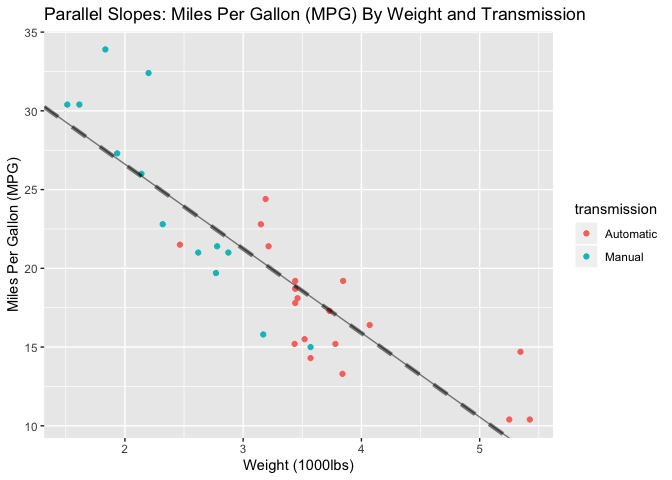Re-assessing the starting questions in light of this model yields very different answers. The confidence intervals for this model if we select the mean weight highlight this. The `mpg` estimates for automatic and manual transmission cars are essentially the same, and for manual cars I need a wider confidence interval.

``````# confidence intervals for automatic and manual transmission cars
ps_ci_a <- predict(ps, newdata = data.frame(transmission = "Automatic",
wt = mean(mtcars\$wt)), interval = "confidence")
ps_ci_m <- predict(ps, newdata = data.frame(transmission = "Manual",
wt = mean(mtcars\$wt)), interval = "confidence")

# arrange in a table
ps_ci <- data.frame(Transmission = c("Automatic", "Manual"),
Lower = c(ps_ci_a, ps_ci_m),
Fit = c(ps_ci_a, ps_ci_m),
Upper = c(ps_ci_a, ps_ci_m))
kable(ps_ci, digits = 3, align = 'c', caption = "Parallel Slopes Model: MPG Confidence Intervals")``````
Parallel Slopes Model: MPG Confidence Intervals
Transmission Lower Fit Upper
Automatic 18.396 20.100 21.804
Manual 17.891 20.077 22.262

In light of this model, the answer to the starting questions would be that the difference between automatic and manual transmission cars in terms of fuel efficiency is essentially 0. Under this model, focusing on transmission is the wrong way of approaching the question. Instead, one should look at the car’s weight in order to predict fuel efficiency.

## Interaction Model

The parallel slopes model allows for different intercepts, but only one slope to explain the relationship amongst the variables. This is most likely an unnatural and definitely an unnecessary restriction. It is possible that the effect that transmission has on `mpg` varies as `wt` varies. For example, perhaps for very heavy cars the choice of transmission is inconsequential, whereas for lighter cars the choice of transmission does have an important impact on fuel economy. An interaction model will allow us to explore this possibility.

``````# interaction model
inter <- lm(mpg ~ wt + transmission + wt:transmission, data = mtcars)
# alternatively: lm(mpg ~ wt * transmission, data = mtcars)
summary(inter)``````
``````##
## Call:
## lm(formula = mpg ~ wt + transmission + wt:transmission, data = mtcars)
##
## Residuals:
##     Min      1Q  Median      3Q     Max
## -3.6004 -1.5446 -0.5325  0.9012  6.0909
##
## Coefficients:
##                       Estimate Std. Error t value Pr(>|t|)
## (Intercept)            31.4161     3.0201  10.402 4.00e-11 ***
## wt                     -3.7859     0.7856  -4.819 4.55e-05 ***
## transmissionManual     14.8784     4.2640   3.489  0.00162 **
## wt:transmissionManual  -5.2984     1.4447  -3.667  0.00102 **
## ---
## Signif. codes:  0 '***' 0.001 '**' 0.01 '*' 0.05 '.' 0.1 ' ' 1
##
## Residual standard error: 2.591 on 28 degrees of freedom
## Multiple R-squared:  0.833,  Adjusted R-squared:  0.8151
## F-statistic: 46.57 on 3 and 28 DF,  p-value: 5.209e-11``````

Here the first and second rows of the coefficient table are the intercept and slope for cars with an automatic transmission, respectively. The third and fourth rows, when added to their counterparts in the first and second rows, give the intercept and slope for cars with a manual transmission.

We also see that R^2 has now raised from 0.75 to 0.83 and all variables yield significant p-values, broadly suggesting that this model is an improvement over the parallel slopes model. Let’s plot this model allowing for different intercepts and slopes amongst manual and automatic transmissions.

``````# plot interaction model
ggplot(mtcars, aes(y = mpg, x = wt, color = transmission)) +
geom_point() +
geom_smooth(method = "lm", se = FALSE) +
labs(y = "Miles Per Gallon (MPG)", x = "Weight (1000lbs)",
title = "Interactive Model: Miles Per Gallon (MPG) By Weight and Transmission")``````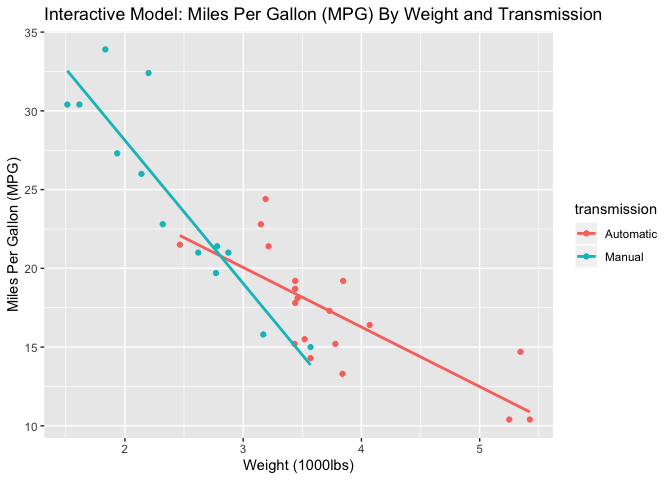By default, R only draws the linear model through the respective range of data, and so we also see here that there is a clear difference in the typical weight of cars by transmission.

This model also suggests that the decrease in fuel efficiency amongst manual transmission cars as weight increases is sharper than that for automatic transmission cars. But we can see that because manual transmission cars tend to be much lighter than automatic transmission cars there is somewhat little overlap in our data.

Given my interest in `transmission`, I am more interested in the model intercepts in this case. Thus far, my answer to the starting question of whether automatic or manual transmission is better for `mpg` depends on knowing the `wt` of the car. According to this interaction model, a car weighing less than approximately 2800 lbs would be expected to have a greater fuel efficiency if it had a manual transmission. However, for a car greater than 2800 lbs, we would expect cars with automatic transmissions to have a higher `mpg` than cars with manual transmissions.

This difference is difficult to quantify with a single number because it changes depending upon the weight. I would expect a large difference in favor of manual transmission cars at very low weights and in favor of automatic cars at very high weights. However, I can examine the difference at the mean car weight of 3217 lbs and build a confidence interval around this point.

``````# confidence intervals for automatic and manual transmission cars
inter_ci_a <- predict(inter,
newdata = data.frame(transmission = "Automatic",
wt = mean(mtcars\$wt)),
interval = "confidence")
inter_ci_m <- predict(inter,
newdata = data.frame(transmission = "Manual",
wt = mean(mtcars\$wt)),
interval = "confidence")

# arrange in a table
inter_ci <- data.frame(Transmission = c("Automatic", "Manual"),
Lower = c(inter_ci_a, inter_ci_m),
Fit = c(inter_ci_a, inter_ci_m),
Upper = c(inter_ci_a, inter_ci_m))
kable(inter_ci, digits = 3, align = 'c', caption = "Interaction Model: MPG Confidence Intervals at Mean Weight")``````
Interaction Model: MPG Confidence Intervals at Mean Weight
Transmission Lower Fit Upper
Automatic 17.729 19.236 20.743
Manual 14.583 17.068 19.553

According to this interaction model, at the mean car weight, we expect a car with an automatic transmission to have a little more than 2 miles per gallon in fuel efficiency over a car with a manual transmission. So overall the parallel slopes and interaction models were a good example as to how adding even one additional predictor variable can completely change our understanding of how two variables are related.

## Multivariable Regression

The parallel slopes and interaction models are useful, particularly for their graphical intuition, but now let’s try adding even more variables that could help explain the relationship behind `mpg` and `transmission`. At the same time, I want the model to remain parsimonious and highly interpretable so I need to be wary of multicollinearity amongst the predictor variables.

A matrix of scatterplots can help give us a sense of what other variables might be useful in predicting `mpg`.

``````# scatterplot matrix
ggpairs(mtcars, columns = 1:7, lower = list(continuous = wrap("smooth", method = "lm")))``````